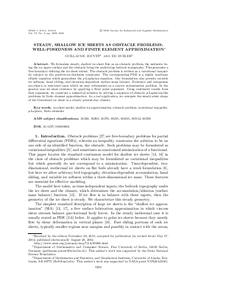Repository: Freie Universität Berlin, Math Department

# Steady, shallow ice sheets as obstacle problems: Well-posedness and finite element approximation

Jouvet, G. and Bueler, E. (2012) Steady, shallow ice sheets as obstacle problems: Well-posedness and finite element approximation. SIAM J. Appl. Math., 72 (4). pp. 1292-1314. ISSN 0036-1399Preview

1MB

Official URL: https://dx.doi.org/10.1137/110856654

## Abstract

We formulate steady, shallow ice sheet flow as an obstacle problem, the unknown being the ice upper surface and the obstacle being the underlying bedrock topography. This generates a free-boundary defining the ice sheet extent. The obstacle problem is written as a variational inequality subject to the positive-ice-thickness constraint. The corresponding PDE is a highly nonlinear elliptic equation which generalizes the \$p\$-Laplacian equation. Our formulation also permits variable ice softness, basal sliding, and elevation-dependent surface mass balance. Existence and uniqueness are shown in restricted cases which we may reformulate as a convex minimization problem. In the general case we show existence by applying a fixed point argument. Using continuity results from that argument, we construct a numerical solution by solving a sequence of obstacle \$p\$-Laplacian-like problems by finite element approximation. As a real application, we compute the steady-state shape of the Greenland ice sheet in a steady present-day climate.

Item Type: Article Mathematical and Computer Sciences > Mathematics > Numerical Analysis Department of Mathematics and Computer Science > Institute of Mathematics 1867 Ekaterina Engel 13 Apr 2016 09:06 03 Mar 2017 14:42

Repository Staff Only: item control page